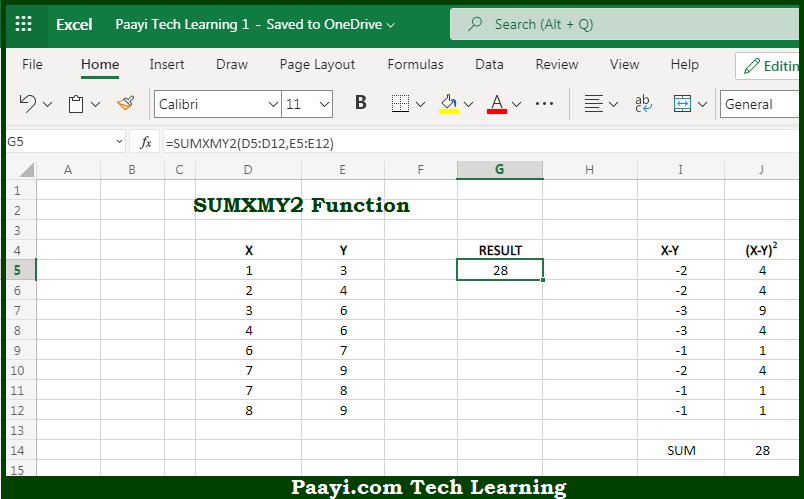# Learn How to Use Microsoft Excel SUMXMY2 Function

Written by | 0 Comments | 669 Views

In this article, you will learn how to use the Microsoft Excel SUMXMY2 function and its prime function in Microsoft Excel. You will also get to know the Microsoft Excel SUMXMY2 function return value and syntax with the help of some examples.

Microsoft Excel SUMXMY2 Function

The main function of the Microsoft Excel SUMXMY2 function is to get the sum of squares of differences in two arrays. That implies, with the help of the SUMXMY2 function you can able to get the sum of the squares of differences between corresponding values in two arrays. It should be noted that the values that you provided can be in the form of constants, cell references, or ranges. So, with the help of the SUMXMY2 function, you can able to get the sum of squares if differences in two arrays.

Return Value of SUMXMY2 Function

The return value will be the calculated sum of squares of differences.

Syntax of SUMXMY2 Function

=SUMXMY2(array-x, array-y)

Where the arguments:

• array-x: This is the first range or array containing numeric values.
• array-y: This is the second range or array containing numeric values (optional).

## How to Use Microsoft Excel SUMXMY2 Function?So we know that Microsoft Excel SUMXMY2 function you can able to get the sum of squares of differences in two arrays. That implies, with the help of the SUMXMY2 function you can able to get the sum of the squares of differences between corresponding values in two arrays. It should be noted that the values that you provided can be in the form of constants, cell references, or ranges. So, with the help of the SUMXMY2 function, you can able to get the sum of squares if differences in two arrays.